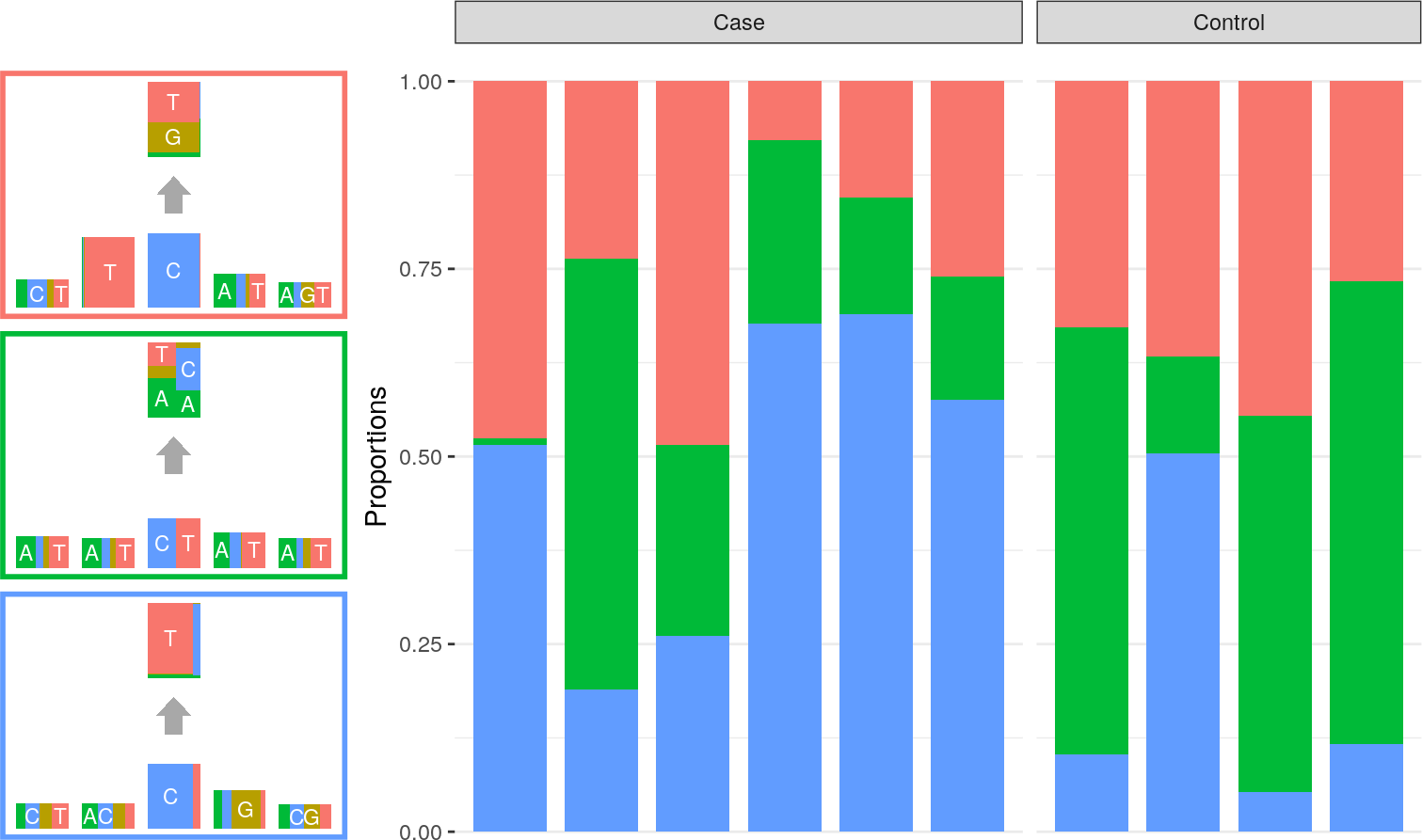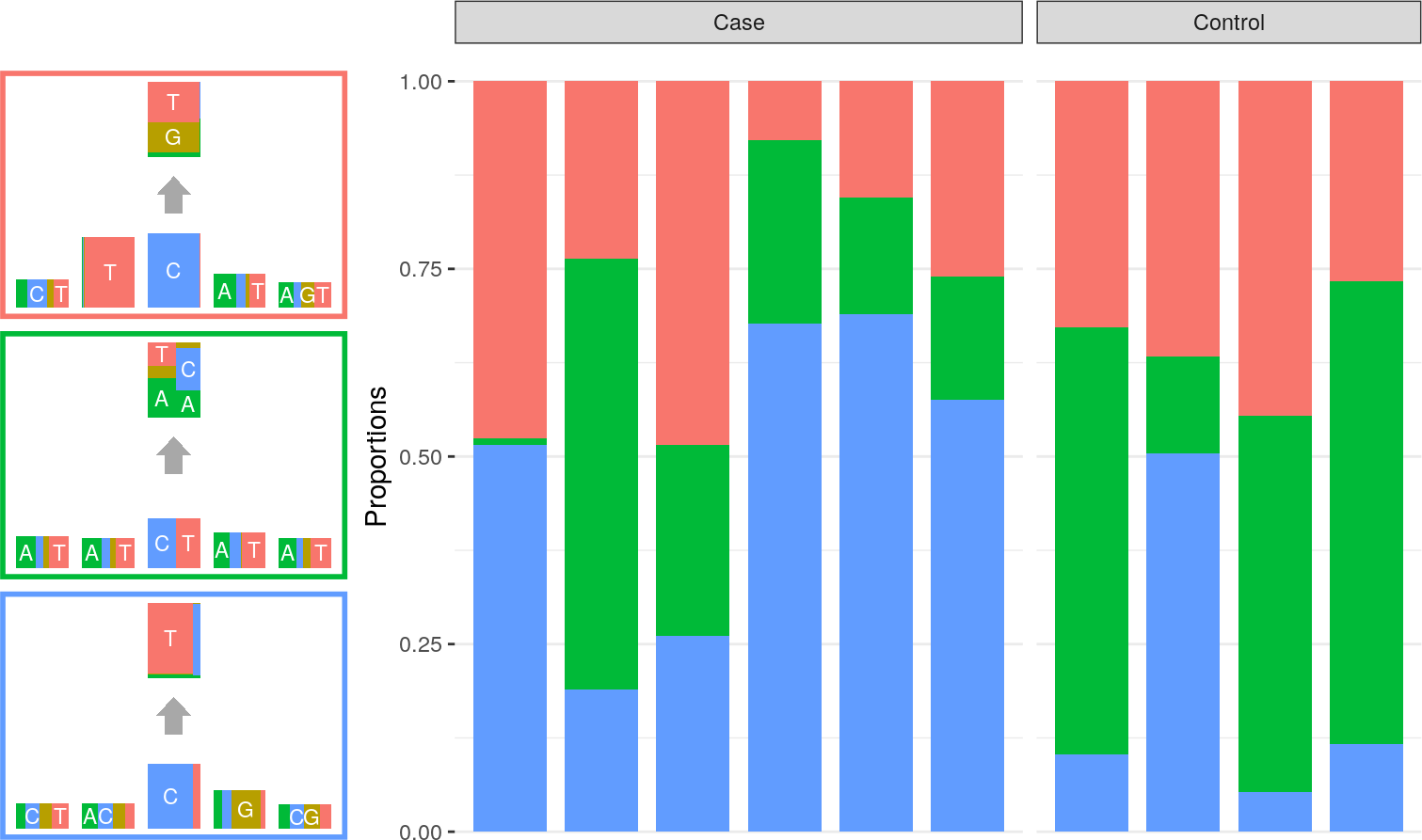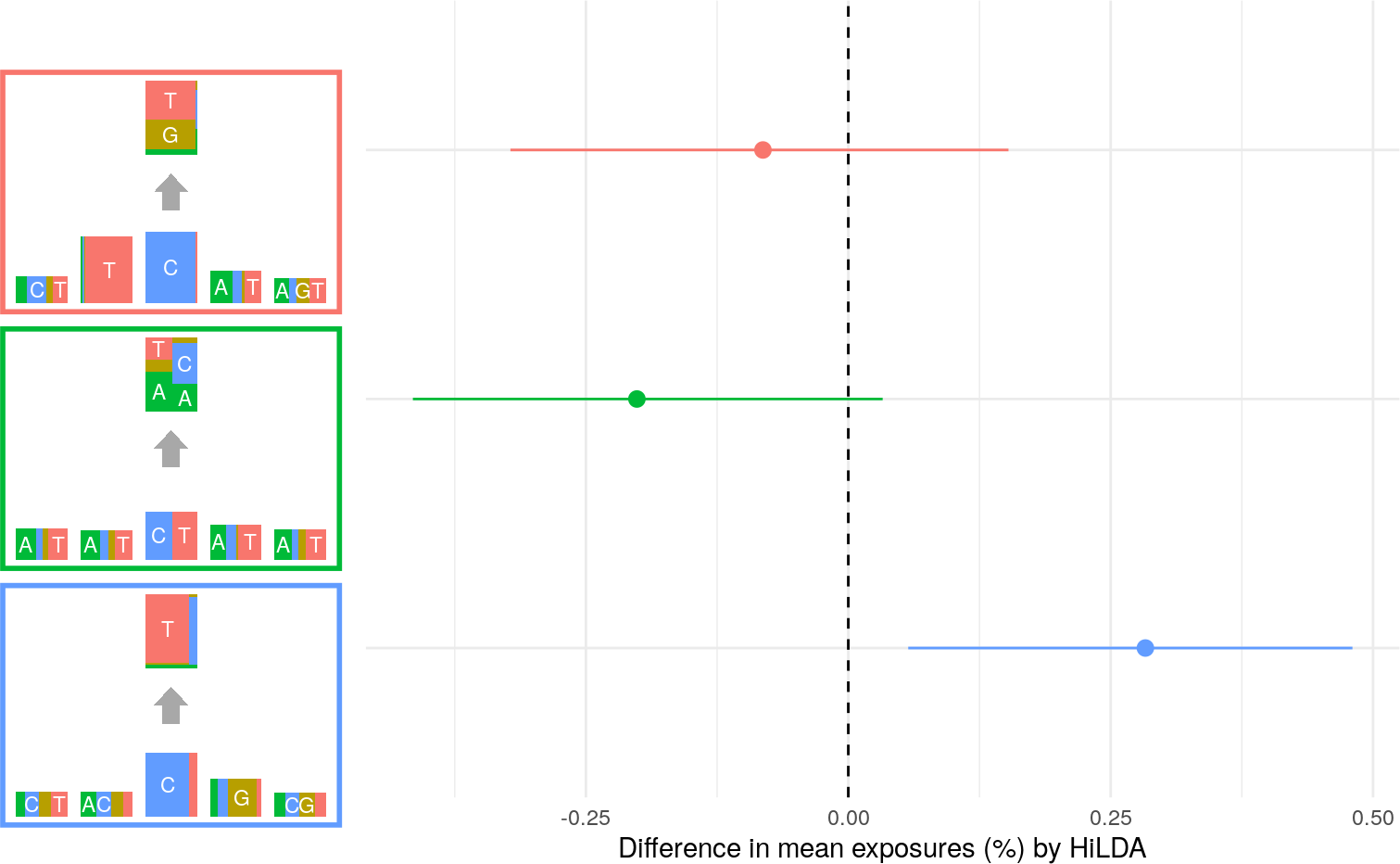# 1 Introduction

The R package HiLDA is developed under the Bayesian framework to allow statistically testing whether there is a change in the mutation burdens of mutation signatures between two groups. The mutation signature is defined based on the independent model proposed by Shiraishi’s et al.

## 1.1 Paper

• Shiraishi et al. A simple model-based approach to inferring and visualizing cancer mutation signatures, bioRxiv, doi: http://dx.doi.org/10.1101/019901.

• Zhi Yang, Priyatama Pandey, Darryl Shibata, David V. Conti, Paul Marjoram, Kimberly D. Siegmund. HiLDA: a statistical approach to investigate differences in mutational signatures, bioRxiv, doi: https://doi.org/10.1101/577452

## 2.1 Installation

### 2.1.1 Bioconductor

HiLDA requires several CRAN and Bioconductor R packages to be installed. Dependencies are usually handled automatically, when installing the package using the following commands:

install.packages("BiocManager")
BiocManager::install("HiLDA")

[NOTE: Ignore the first line if you already have installed the BiocManager.]

devtools::install_github("USCbiostats/HiLDA")

### 2.1.2 Just Another Gibbs Sampler (JAGS)

In order to run HiLDA, one also needs to install an external program called Just Another Gibbs Sampler, JAGS, downloaded from this website http://mcmc-jags.sourceforge.net/. For more details, please follow the INSTALL file to install the program.

# 3 Input data

HiLDA is a package built on some basic functions from pmsignature including how to read the input data. Here is an example from pmsignature on the input data, mutation features are elements used for categorizing mutations such as:

• 6 substitutions (C>A, C>G, C>T, T>A, T>C and T>G)
• 2 flanking bases (A, C, G and T)
• transcription direction.

## 3.1 Mutation Position Format

sample1 chr1  100   A   C
sample1 chr1    200 A   T
sample1 chr2    100 G   T
sample2 chr1    300 T   C
sample3 chr3    400 T   C   
• The 1st column shows the name of samples
• The 2nd column shows the name of chromosome
• The 3rd column shows the coordinate in the chromosome
• The 4th column shows the reference base (A, C, G, or T).
• The 5th colum shows the alternate base (A, C, G, or T).

# 4 Workflow

## 4.1 Get input data

Here, inputFile is the path for the input file. numBases is the number of flanking bases to consider including the central base (if you want to consider two 5’ and 3’ bases, then set 5). Also, you can add transcription direction information using trDir. numSig sets the number of mutation signatures estimated from the input data. You will see a warning message on some mutations are being removed.

library(HiLDA)
## Loading required package: ggplot2
inputFile <- system.file("extdata/esophageal.mp.txt.gz", package="HiLDA")
G <- hildaReadMPFile(inputFile, numBases=5, trDir=TRUE)
## Warning in hildaReadMPFile(inputFile, numBases = 5, trDir = TRUE): Out of
## 24861 mutations, we could obtaintranscription direction information for 24728
## mutation. Other mutations are removed.

Also, we also provided a small simulated dataset which contains 10 mutational catalogs andused it for demonstrating the key functions in HiLDA. We start with loading the sample dataset G stored as extdata/sample.rdata.

load(system.file("extdata/sample.rdata", package = "HiLDA"))
class(G)
##  "MutationFeatureData"
## attr(,"package")
##  "HiLDA"

## 4.2 Run tests from HiLDA

### 4.2.1 Perform the global test and the local test

After we read in the sample data G, we can run the local and the global tests from HiLDA. Here, we specify the inputG as G, the number of mutational signatures to be three, the indices for the reference group to be 1:4, the number of iterations to be 1000. localTest being FALSE means that a global test is called while it being TRUE means that a local test is called instead.

set.seed(123)
hildaGlobal <- hildaTest(inputG=G, numSig=3, localTest=FALSE,
refGroup=1:4, nIter=1000)
## module glm loaded
## Compiling model graph
##    Resolving undeclared variables
##    Allocating nodes
## Graph information:
##    Observed stochastic nodes: 6370
##    Unobserved stochastic nodes: 1304
##    Total graph size: 14909
##
## Initializing model
hildaLocal <- hildaTest(inputG=G, numSig=3, localTest=TRUE,
refGroup=1:4, nIter=1000)
## Compiling model graph
##    Resolving undeclared variables
##    Allocating nodes
## Graph information:
##    Observed stochastic nodes: 6370
##    Unobserved stochastic nodes: 1305
##    Total graph size: 14903
##
## Initializing model

## 4.3 Get signatures from pmsignature

This object is used to provide an initial values for running MCMC sampling to reduce the running time by using the EM algorithm from pmsignature package developed by Shiraishi et al.

Param <- pmgetSignature(G, K = 3)
## #trial:  1; #iteration:   64; time(s): 0.08; convergence: TRUE; loglikelihood: -8202.3184
## #trial:  2; #iteration:   27; time(s): 0.03; convergence: TRUE; loglikelihood: -8202.3184
## #trial:  3; #iteration:   39; time(s): 0.05; convergence: TRUE; loglikelihood: -8202.3200
## #trial:  4; #iteration:   21; time(s): 0.03; convergence: TRUE; loglikelihood: -8202.3187
## #trial:  5; #iteration:   63; time(s): 0.08; convergence: TRUE; loglikelihood: -8202.3185
## #trial:  6; #iteration:   12; time(s): 0.02; convergence: TRUE; loglikelihood: -8202.3184
## #trial:  7; #iteration:   22; time(s): 0.05; convergence: TRUE; loglikelihood: -8202.3334
## #trial:  8; #iteration:   22; time(s): 0.05; convergence: TRUE; loglikelihood: -8202.3184
## #trial:  9; #iteration:   12; time(s): 0.03; convergence: TRUE; loglikelihood: -8202.3184
## #trial: 10; #iteration:   20; time(s): 0.04; convergence: TRUE; loglikelihood: -8202.3187

### 4.3.1 Perform the global test and the local test with initial values

In a very similar way as running the HiLDA test, one just needs to specify useInits to be Param returned by the previous function to allow the initial values to be used in the MCMC sampling.

set.seed(123)
hildaGlobal <- hildaTest(inputG=G, numSig=3, useInits = Param,
localTest=TRUE, refGroup=1:4, nIter=1000)
## Compiling model graph
##    Resolving undeclared variables
##    Allocating nodes
## Graph information:
##    Observed stochastic nodes: 6370
##    Unobserved stochastic nodes: 1305
##    Total graph size: 14903
##
## Initializing model
hildaLocal <- hildaTest(inputG=G, numSig=3, useInits = Param,
localTest=TRUE, refGroup=1:4, nIter=1000)
## Compiling model graph
##    Resolving undeclared variables
##    Allocating nodes
## Graph information:
##    Observed stochastic nodes: 6370
##    Unobserved stochastic nodes: 1305
##    Total graph size: 14903
##
## Initializing model

### 4.3.2 Assess Convergence of MCMC chains

After the MCMC sampling finishes, we can compute the potential scale reduction statistic to examine the convergence of two chains. Usually it is recommended to be less than 1.10. If not, it can be done by increasing the number of nIter.

hildaRhat(hildaGlobal)
##  1.05479
hildaRhat(hildaLocal)
##  1.147583

## 4.4 Visualize the mutation signatures from pmsignature

To allow users to compare the mutational signatures from both pmsignature and HiLDA, this function is used to plot the results from pmsignature.

pmPlots <- pmBarplot(G, Param, refGroup=1:4, sigOrder=c(1,3,2))
cowplot::plot_grid(pmPlots$sigPlot, pmPlots$propPlot, rel_widths = c(1,3))## 4.5 Visualize the mutation signatures from HiLDA

In contrast, the following function is used to plot the results from HiLDA.

hildaPlots <- hildaBarplot(G, hildaLocal, refGroup=1:4, sigOrder=c(1,3,2))
cowplot::plot_grid(pmPlots$sigPlot, pmPlots$propPlot, rel_widths = c(1,3))## 4.6 Output the posterior distribution of the mean difference in exposures

To visualize the 95% credible interval of the mean differences in exposures, the following function plots the differences along with the mutational signatures.

hildaDiffPlots <- hildaDiffPlot(G, hildaLocal, sigOrder=c(1,3,2))
cowplot::plot_grid(hildaDiffPlots$sigPlot, hildaDiffPlots$diffPlot,
rel_widths = c(1,3))